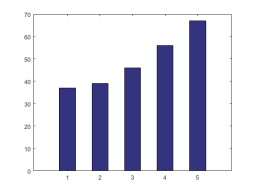# The midpoint 3

Ranges are 10-20 20-30 30-40 40-50. If the frequency is 7 15 26 12, what is the midpoint?

x =  32.1667

### Step-by-step explanation:Did you find an error or inaccuracy? Feel free to write us. Thank you!

Tips for related online calculators
Looking for help with calculating arithmetic mean?
Looking for a statistical calculator?
Need help calculating sum, simplifying, or multiplying fractions? Try our fraction calculator.

#### Grade of the word problem:

We encourage you to watch this tutorial video on this math problem: